• Mood:
• Music:

# [icons] major haul!

It's been a while since I've done a proper, non-challenge update, I thought it was due.

Nothing really special to say about this latest batch of icons - apart from there's a lot of them, and they're made using the same method. So if you like them and want to know how they're created, drop me a message. I may do a tutorial in the future.

 X/1999
 Gekka Kajin Lumen Lunae
 Card Captor Sakura
 Ludwig Kakumei
 Angel Sanctuary
 DNAngel
 Bloodhound
 Fafner
 RG Veda
 Sailormoon
=53 icons

Remember: please comment, and credit my icons if you plan to use them (either or ). Thank you~

.teasers.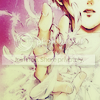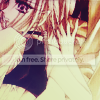X/1999

 =01= =02= =03=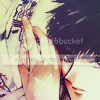=04= =05= =06=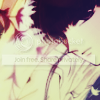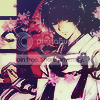=07= =08= =09=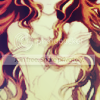=10= =11= =12=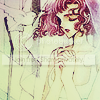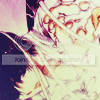=13= =14= =15=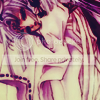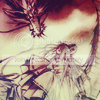= =16= =GEKKA KAJIN LUMEN LUNAE

 =17= =18= =19=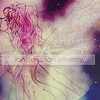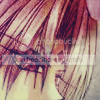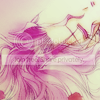=20= =23= =22=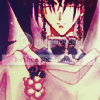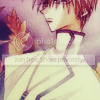=23= =24= =25=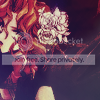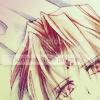= =26= =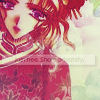CARDCAPTOR SAKURA

 =27= =28= =29=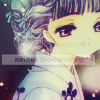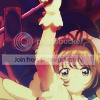=30= =31= =32=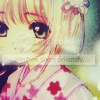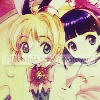=33= =34= =35=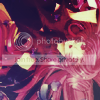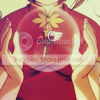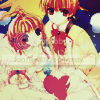LUDWIG KAKUMEI

 =36= =37= =38==39= =40= =41=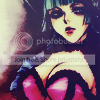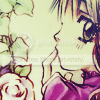ANGEL SANCTUARY

 =42= =43= =44=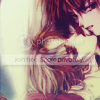=045= = =46=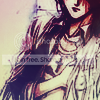DNANGEL

 =47= =48= =49=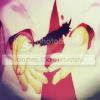MISC ICONS

 =50= =51= =52=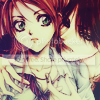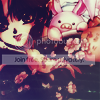= =53= =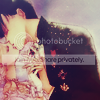• #### [tutorial] setsuna - deepened colours

Since I had a nice response to my last icon post (thanks guys!), which used this particular method to make the icons, I have relented and decided to…

• #### [tutorial] another bunch of icon tutorials!

I haven't done a tutorial post for quite a while, and here I am presenting two! Wow. And I've decided to show how the effects I gain look on other…

• #### [tutorial] sakura - easiest tutorial ever?

Incredibly easy tutorial today, yet delivers a really nice result. I was actually asked how to make this on my personal journal a while ago, but have…

• Post a new comment

#### Error

default userpic
When you submit the form an invisible reCAPTCHA check will be performed.
You must follow the Privacy Policy and Google Terms of use.
• 21 comments
Previous
← Ctrl ← Alt
Next
Ctrl → Alt →
Previous
← Ctrl ← Alt
Next
Ctrl → Alt →
• #### [tutorial] setsuna - deepened colours

Since I had a nice response to my last icon post (thanks guys!), which used this particular method to make the icons, I have relented and decided to…

• #### [tutorial] another bunch of icon tutorials!

I haven't done a tutorial post for quite a while, and here I am presenting two! Wow. And I've decided to show how the effects I gain look on other…

• #### [tutorial] sakura - easiest tutorial ever?

Incredibly easy tutorial today, yet delivers a really nice result. I was actually asked how to make this on my personal journal a while ago, but have…